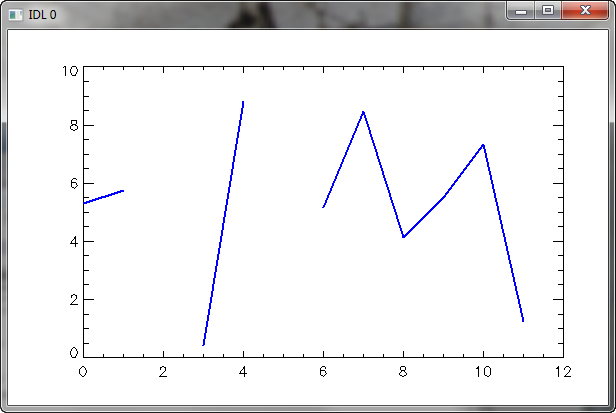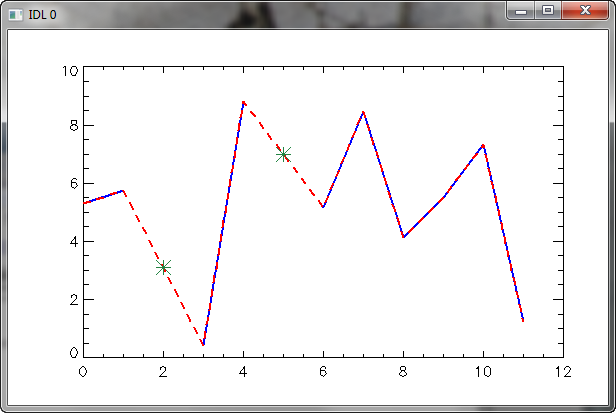Interpolating Bad Data Points in a Vector

QUESTION: I have a data vector with some bad data points in it. I would like to remove these points are replace them with points that are interpolated from the good data points in the vector. Is there an easy way to do this in IDL?ANSWER: Yes, the IDL INTERPOL command can be used for this purpose. Consider the following example provided by JD Smith, in which the "bad" data is represented by values greater than 10.

IDL> vector = RandomU(-3L, 12) * 10
IDL> vector[[2,5]] = [15, 25]
IDL> Print, vector, Format='(12(F5.2,x))'
8.98  5.58 15.00  5.89  0.60 25.00  0.38  2.18  1.42  9.85  8.95  9.48
IDL> cgPlot, vector, Max_Value=10, Color='blue'The vector plotted with the "bad" data screened out with the Max_Value keyword.

First, find the locations of the "bad" data, as well as the locations of the good data, and the number of each.

The interpolation of the data, using INTERPOL, is straighforward:The non-interpolated (blue) and interpolated (red) vectors, with the interpolated points shown in green.